GFG App
Open AppBrowser
Continue

# Class 9 NCERT Solutions- Chapter 14 Statistics – Exercise 14.3

### Question 1. A survey conducted by an organization for the cause of illness and death among the women between the ages 15–44 (in years) worldwide, found the following figures (in %):

(i) Represent the information given above graphically.

(ii) Which condition is the major cause of women’s ill health and death worldwide?

(iii) Try to find out, with the help of your teacher, any two factors which play a major role in the cause in (ii) above being the major cause.

Solution:

(i) The information given in the question can be represented graphically as: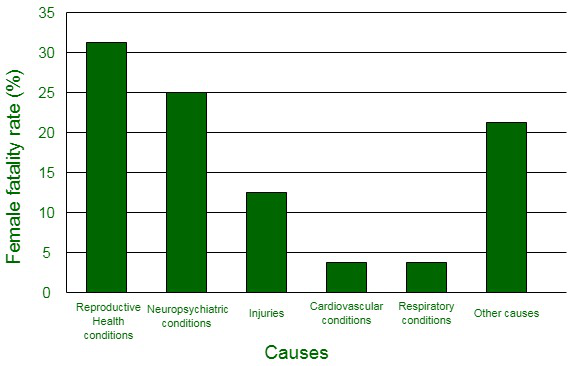(ii) It can be observed from the graph that the reproductive health conditions is the major cause of women’s ill health and death worldwide.

(iii) Two factors which play a major role in the cause in (ii) above being the major cause are:

• Lack of proper care and understanding.
• Lack of medical facilities.

### Question 2. The following data on the number of girls (to the nearest ten) per thousand boys in different sections of Indian society is given below:

(i) Represent the information above by a bar graph.

(ii) In the classroom discuss what conclusions can be arrived at from the graph.

Solution:

(i) The information given in the question can be represented graphically as: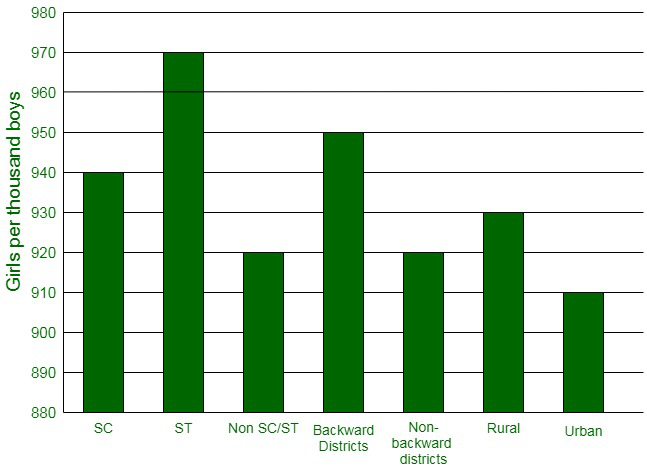(ii) The following conclusions can be made from the graph,

• The maximum number of girls per thousand boys is present in the section ST.
• The backward districts and rural areas have more number of girls per thousand boys than non-backward districts and urban areas.

### Question 3. Given below are the seats won by different political parties in the polling outcome of a state assembly elections:

(i) Draw a bar graph to represent the polling results.

(ii) Which political party won the maximum number of seats?

Solution:

(i) The bar graph representing the polling results is given below: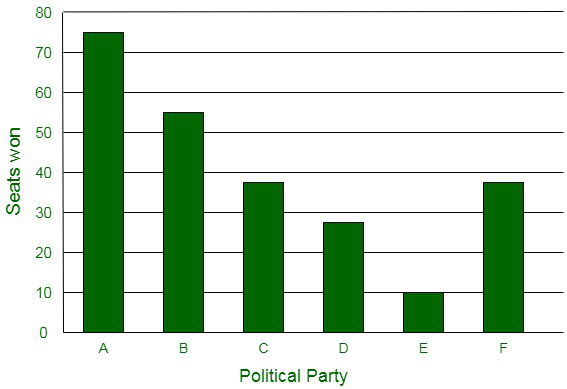(ii) It can be easily visualized that Party A won the maximum number of seats.

### Question 4. The length of 40 leaves of a plant are measured correct to one millimeter, and the obtained data is represented in the following table:

(i) Draw a histogram to represent the given data. [Hint: First make the class intervals continuous]

(ii) Is there any other suitable graphical representation for the same data?

(iii) Is it correct to conclude that the maximum number of leaves are 153 mm long? Why?

Solution:

(i) The data given in the question is represented in discontinuous class interval. In order to make it in a continuous class interval., we subtract ½ = 0.5 from lower limit and add 0.5 to the upper limit. Then, the table becomes: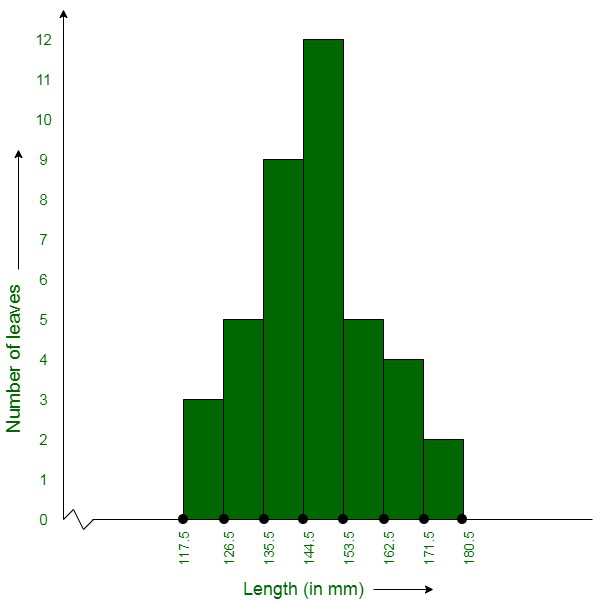(ii) Yes, the data given in the question can also be represented in the form of a frequency polygon.

No, we cannot conclude that the maximum number of leaves are 153 mm long because the maximum number of leaves are lying in-between the length of 144.5 – 153.5

### Question 5. The following table gives the life times of 400 neon lamps:

(i) Represent the given information with the help of a histogram.

(ii) How many lamps have a lifetime of more than 700 hours?

Solution:

(i) The histogram representation of the given data is given below: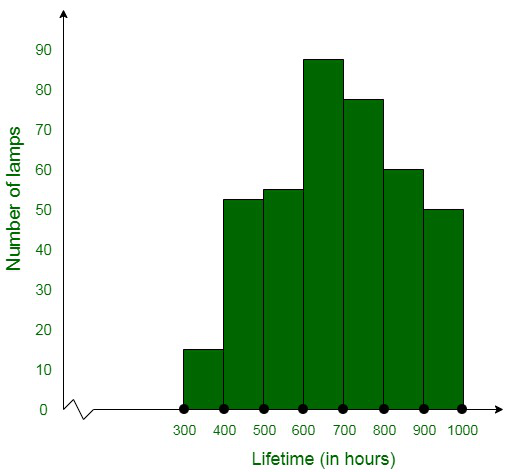(ii) The total number of lamps having a lifetime of more than 700 hours = 74+62+48

= 184

### Question 6. The following table gives the distribution of students of two sections according to the marks obtained by them:

Represent the marks of the students of both the sections on the same graph by two frequency polygons. From the two polygons compare the performance of the two sections.

Solution:

The class-marks = (lower limit + upper limit)/2

Section A:

Section B:

Representing these data on a graph using two frequency polygons we get,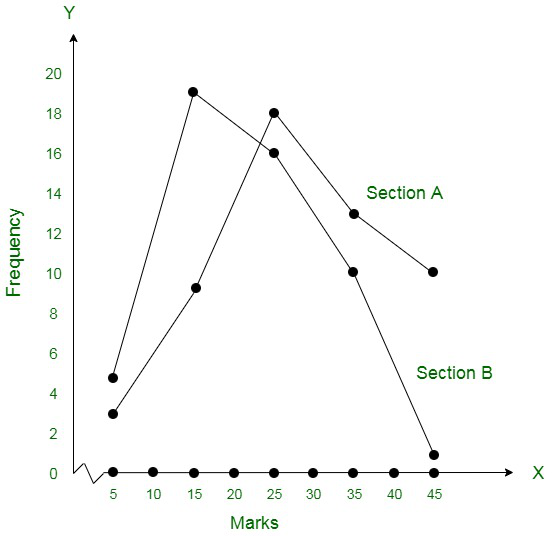Conclusively, the students of Section A performed better than Section B.

### Question 7. The runs scored by two teams A and B on the first 60 balls in a cricket match are given below:

Represent the data of both the teams on the same graph by frequency polygons.

[Hint: First make the class intervals continuous.]

Solution:

The data given in the question is represented in discontinuous class interval. In order to make it in continuous class interval, we subtract ½ = 0.5 = 0.5 from lower limit and add 0.5 to the upper limit. Then the table becomes:

The data of both the teams are represented on the graph below by frequency polygons.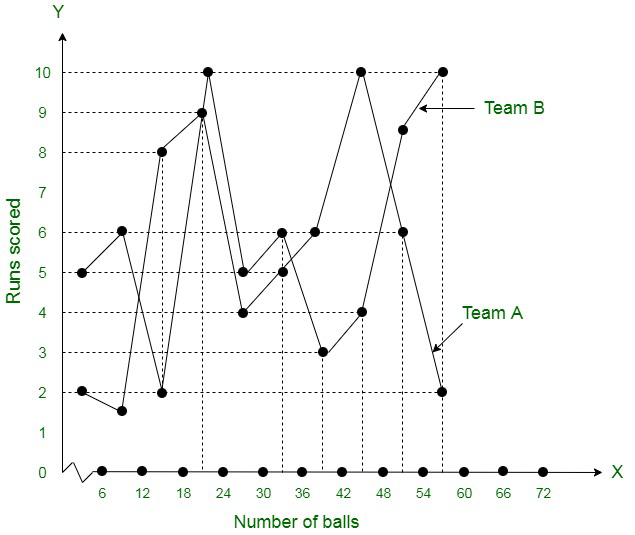### Question 8. A random survey of the number of children of various age groups playing in a park was found as follows:

Draw a histogram to represent the data above.

Solution:

The width of the class intervals in the given data is varying in nature.

Also,

The area of rectangle is proportional to the frequencies in the histogram.

Now, the proportion of the children per year can be calculated as given in the table below.

Let us assume,

x-axis = the age of children

y-axis = proportion of children per 1 year interval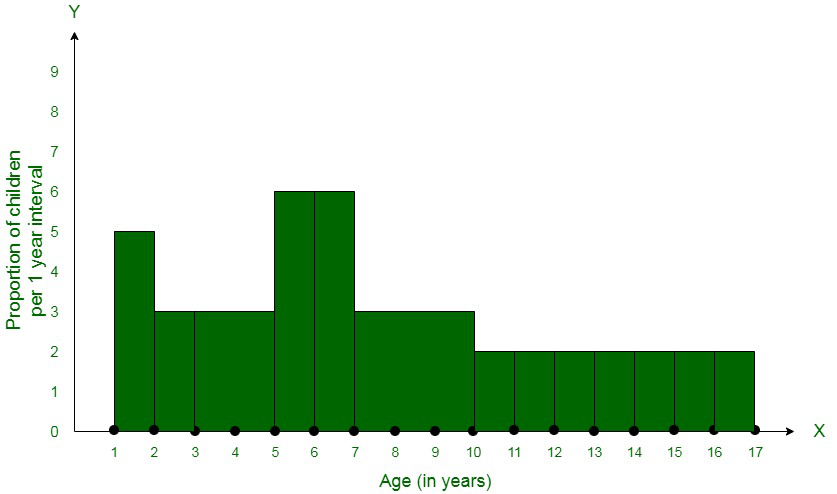### Question 9. 100 surnames were randomly picked up from a local telephone directory and a frequency distribution of the number of letters in the English alphabet in the surnames was found as follows:

(i) Draw a histogram to depict the given information.

(ii) Write the class interval in which the maximum number of surnames lie.

Solution:

(i) The width of the class intervals in the given data is varying in nature.

Also,

The area of rectangle is proportional to the frequencies in the histogram.

Now, the proportion of the children per year can be calculated as given in the table below.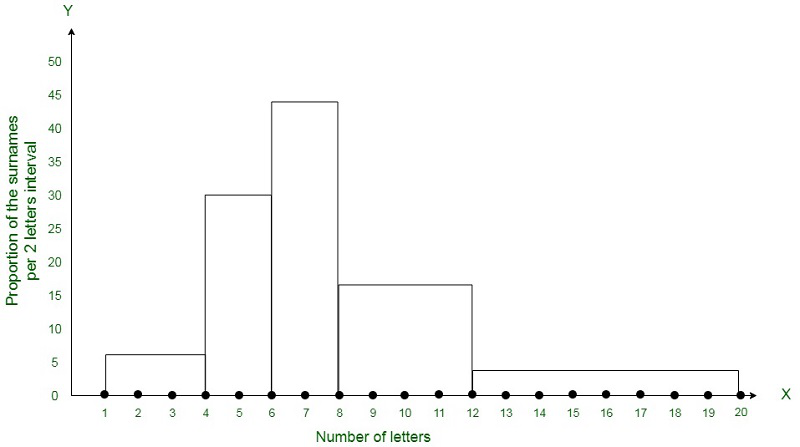(ii) 6-8 is the class interval in which the maximum number of surnames lie.

My Personal Notes arrow_drop_up